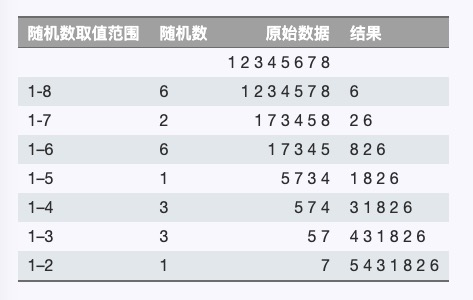# Fisher–Yates shuffle 洗牌算法

《Statistical tables for biological, agricultural and medical research》书中给出的算法描述

1964 年由 Richard Durstenfeld 改编为适用于电脑编程的版本

n - 1 上的数与 n - 2 个随机位置上的数交换## # 多版本对比

「FY」（洗牌算法）原理相当简单

### # lodash 版本

「lodash」是前端开发常用的工具类库

``````function shuffle(array) {
const length = array == null ? 0 : array.length
if (!length) {
return []
}
let index = -1 // 待交换索引位，从数组第 0 项开始
const lastIndex = length - 1
const result = copyArray(array)
while (++index < length) {
// 随机索引位，将[0, length - 1] 中区间中取出随机整数
const rand = index + Math.floor(Math.random() * (lastIndex - index + 1))
// 取出随机索引位值，此时随机索引位就空出来了
const value = result[rand]
// 使用待交换索引位值填充空出来的随机索引位
result[rand] = result[index]
// 将之前取出的随机索引位值赋值给待交换索引位
result[index] = value
}
return result
}

export default shuffle
``````
1
2
3
4
5
6
7
8
9
10
11
12
13
14
15
16
17
18
19
20
21
22

lodash 中 shuffle 源码 (opens new window)

• lodash 版本多了关于边界的异常处理，比如数组长度为 0 时不进行直接返回

• 从数组起始 0 位置索引开始交换

• 遍历的次数是`数组的长度 - 1`

• 随机索引位与待交换索引位可能重复，比如 index 与 rand 值都可能为 1

### # phaser3 版本

「phaser3」 是一款游戏开发引擎，其工具类对象中也有关于此的实现

``````var Shuffle = function (array)
{
// 缺少 i = 0 时的交换
for (var i = array.length - 1; i > 0; i--)
{
var j = Math.floor(Math.random() * (i + 1));
var temp = array[i];
array[i] = array[j];
array[j] = temp;
}

return array;
};

module.exports = Shuffle;
``````
1
2
3
4
5
6
7
8
9
10
11
12
13
14
15

phash3 中 shuffle 源码 (opens new window)

• 从数组最大索引位置开始交换

• 无异常处理

• 遍历次数为 `数组长度 - 2` 次，缺少一次遍历，最后一次遍历数组只有一项，随机索引与待交换索引相同，交换无意义，结果不影响

• 随机索引位与待交换索引位可能重复

## # 扩展 Array 方法

``````Array.prototype.shuffle = function() {
// 当 [1, 2, 3, 4].shuffle() 调用时
// this = [1, 2, 3, 4]
let input = this
for (let i = input.length - 1; i >= 0; i --) {
let rand = Math.floor(Math.random() * (i + 1))
let valAtRand = input[rand]
input[rand] = input[i]
input[i] = valAtRand
}
return input
}

// 使用
let arr = [1, 2, 3, 4]
arr.shuffle() // [4, 1, 2, 3]，结果是随机的，所以看到的与此不一致正常
``````
1
2
3
4
5
6
7
8
9
10
11
12
13
14
15
16

## # 小结